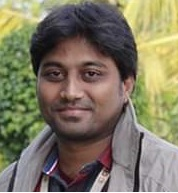# CUNT2481 Integral EquationTeacher

##### A AvinashCategory

### Course Attendees

Still no participant

### Course Reviews

Still no reviews

Course outline
CUNT2481   Integral Equation

 Subject Name Code Type of course T-P-Pj Prerequisite Integral Equation CUNT2481 Theory  & Project 3-0-1 NIL

Objective

 This course unit aims to identify different type of Integral equations  To Solve different type of Boundary value problems of Integral Equations in nature To Covert differential equations into Integral equations.

Learning outcome

 Upon successful completion of this course, students will be able to: Solve Integral equations of different types. Solve Boundary Value  Problems of Integral type

Course outline

### Introduction, Definitions of Integral Equation ,Linear, Non Linear Equations ,Fredholm Integral Equation, Volterra Integral Equation, Singular Integral Equation, Special  Kinds of Kernels , Integral equations of Convolution type, Iterated Kernel sand Resolvent  Kernel.

Project 1: Prepare a detail report of different kind of Integral Equations.

### Module-II

Eigen values, Leibnitz’s rule of differentiation under integral sign, Formula for converting  multiple integral into single ordinary integral, Regularity conditions, Inner product of two functions, Definition and  some simple examples of Solution of Integral Equations.

Project 2: Prepare a report on Leibnitz’s rule of differentiation under integral sign and
Formula for converting multiple integral into single ordinary integral.

### Module-III

Conversion of Ordinary differential equations into integral equations.

Project 3: Prepare a report on advantages of  Conversion of Ordinary differential equations
into integral equations

### Module-IV

Homogeneous Fredholm Integral Equations of the Second kind with Separable Kernels.
Project-4 : Prepare a report on advantages of  Fredholm Integral Equations of the Second kind.

### Module-VII

Solution of Volterra Integral Equation of the Second Kind  by Successive Substitutions.

Project-6: Prepare a report on Solution of Volterra Integral Equation of the Second Kind  by
Successive Substitutions

BOOK PRESCRIBED

1. Integral Equations and Boundary Value Problems by M.D. Raisinghania, S.Chand & Company pvt Ltd.  Ch-1, Ch-2, Ch-3, Ch-4 Ch-5 (5.1-5.7)

BOOK FOR REFERENCE

1. Introduction to Integral Equations with Applications , A.J. Jerri, Wiley-Interscience Publication,1999
2. Linear Integral Equations, W.V  Lovitt, , McGraw Hill, New York

Session-1:

Introduction, Definitions of Integral Equation, Linear, Non Linear Equation ,

Session-2

Fredholm Integral Equation, Volterra Integral Equation, Singular Integral Equation

Session-3

Special Kinds of Kernels , Integral equations of Convolution type

Session-4

Iterated Kernels and Resolvent Kernel.

Session-5 & 6:

Project 1: Prepare a detail report of different kind of Integral Equations

Session-7

Eigen values, Leibnitz’s rule of differentiation under integral sign

Session-8

Formula for converting multiple integral into single ordinary integral

Session-9

Regularity conditions, Inner product of two functions

Session-10

Definition and some simple examples of Solution of Integral Equations.

Session-11 &12

Project 2: Prepare a report on Leibnitz’s rule of differentiation under integral sign and Formula for converting multiple integral into single ordinary integral.

Session-13

Conversion of Ordinary differential equations into integral equations.

Session-14

Conversion of Ordinary differential equations into integral equations.

Session-15

Conversion of Ordinary differential equations into integral equations.

Session-16

Conversion of Ordinary differential equations into integral equations.

Session- 17 & 18

Project 3: Prepare a report on advantages of Conversion of Ordinary differential equations

Session Plan:

Session Plan:

Session Plan:

Session Plan:

Session Plan:

Session Plan:

Session Plan:

Session Plan:

Session Plan:

Session Plan:

Session Plan:

Session Plan:

Session Plan:

Session Plan:

Session Plan:

Session Plan:

Session Plan:

Session Plan:

Session Plan:

### Our Main Teachers### A Avinash

##### Asst Professor
VIEW PROFILE

A.Avinash working as Assistant Professor, Dept of CSE, Centurion University of Technology and Management, Andhra Pradesh . Interested to work on Machine learning,Natural Language Processing,Problem Solving Methodologies , and ChatBot. Programming Skill: C Programming Data Structure Object Oriented Programming using C++ Formal Language Automata Theory Python Web Development(HTML,CSS,PHP) Database Management Systems Compilers